## Laminar viscous compressible compression corner flow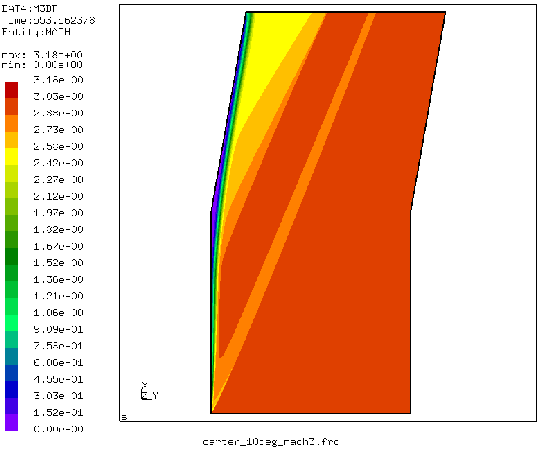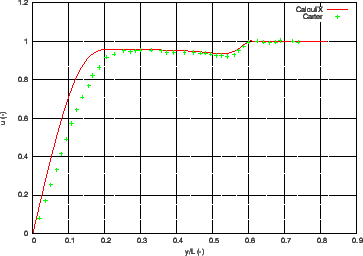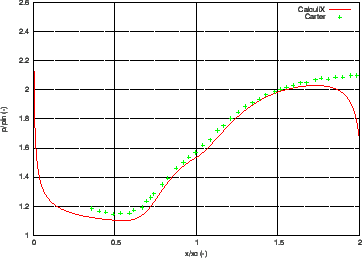This benchmark example is described in . The input deck for the CalculiX computation is called carter_10deg_mach3.inp and can be found in the fluid test example suite. The flow is entering at Mach 3 parallel to a plate of length 16.8 after which a corner of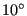arises. The Reynolds number based on a unit length is 1000., which yields for a unit velocity a dynamic viscosity coefficient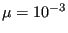. No units are specified: the user can choose appropriate consistent units. Choosing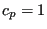and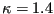leads to a specific gas constant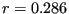. The selected Mach number leads to an inlet temperature of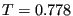. The ideal gas law yields a static inlet pressure of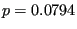(assuming an unit inlet density). The wall is assumed to be isothermal at a total temperature of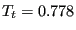. Finally, the assumed Prandl number (Pr=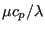) of 0.72 leads to a conduction coefficient of 0.00139.

A very fine mesh with about 425,000 nodes was generated, gradually finer towards the wall (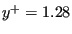for the closest node near the wall at L=1 from the inlet). The Mach number is shown in Figure 32. The shock wave emanating from the front of the plate and the separation and reattachment compression fan at the kink in the plate are cleary visible. One also observes the thickening of the boundary layer near the kink leading to a recirculation zone. Figure 33 shows the velocity component parallel to the inlet plate orientation across a line perpendicular to a plate at unit length from the entrance. One notices that the boundary layer in the CalculiX calculation is smaller than in the Carter solution. This is caused by the temperature-independent viscosity. Applying the Sutherland viscosity law leads to the same boundary layer thickness as in the reference. In CalculiX, no additional shock smoothing was necessary. Figure 34 plots the static pressure at the wall relative to the inlet pressure versus a normalized plate length. The reference length for the normalization was the length of the plate between inlet and kink (16.8 unit lengths). So the normalized length of 1 corresponds to the kink. There is a good agreement between the CalculiX and the Carter results, apart from the outlet zone, where the outlet boundary conditions influence the CalculiX results.